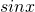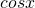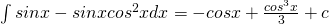# Integrating Trigonometric functions (part 1)Integration is topic that eludes several students. Many think that its those “you either see or don’t” topic. But its all practice and a bit of tricks. Let me touch on integrating trigonometric functions first and we shall start withEasy!This requires double angle formula:Here we introduce trigo identity:Here we have a problem!Notice thatis the derivative () of.

So.

Finally,Here we can apply double angle a few times to break it down before integrating.

So if you notice, this is essentially like an algorithm, and as the power increases the treatment is really quite similar.

Let’s look atin the my next post!

• […] Integrating Trigonometric Function #1 Integrating Trigonometric Function #2 Integrating Trigonometric Function #3 Integrating Trigonometric Function #4 Integrating Trigonometric Function #5 […]

• […] How to derive the sum to produce formula 2. Integrating Trigonometric Functions (1) 3. Integrating Trigonometric Functions (2) 4. Integrating Trigonometric Functions (3) 5. […]

• […] Integrating Trigonometric Functions (1) 2. Integrating Trigonometric Functions (2) 3. Integrating Trigonometric Functions (3) 4. […]

Not readable? Change text.